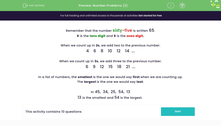# Solve Number Problems (2)

In this worksheet, students will practise place value and number facts to solve a variety of problems.Key stage:  KS 1

Curriculum topic:   Number: Number and Place Value

Curriculum subtopic:   Solve Place Value Problems to 100

Difficulty level:#### Worksheet Overview

This activity is all about numbers.Remember that the number sixty-five is written 65.

6 is the tens digit and 5 is the ones digit.

So the number 65 has 6 tens (60) and 5 ones.

How many tens and how many ones are in the number 59?

There are 5 tens (50) and 9 ones.

What number do we make if we have 4 tens and 3 ones?

It makes the number 4(forty-three)

When we count up in 2s, we add two to the previous number.

4    6    8    10    12    14  ...

What number would come next? 14 + 2 = 16

The sequence above includes only even numbers.

When we count up in 3s, we add three to the previous number.

6    9    12    15    18    21  ...

What number would come next? 21 + 3 = 24

The sequence above is actually part of the three times table.In a list of numbers, the smallest is the one we would say first when we are counting up.

The largest is the one we would say last.

These numbers have all been jumbled up out of order: 45, 34, 25, 54, 13

13 is the smallest and 54 is the largest.

If you arranged them all in order they would go: 13, 25, 34, 45, 54

You need to look at the number in the tens column first when arranging them in order. The largest number (54) has 5 tens and the smallest number (13) has only 1 ten.

Have a look at these numbers: 71, 69, 12, 43, 55

If we arrange them in order, we get: 12, 43, 55, 69, 71. So 12 was the smallest number and 71 was the largest.

Are you ready to have a go at some questions now?### What is EdPlace?

We're your National Curriculum aligned online education content provider helping each child succeed in English, maths and science from year 1 to GCSE. With an EdPlace account you’ll be able to track and measure progress, helping each child achieve their best. We build confidence and attainment by personalising each child’s learning at a level that suits them.

Get started